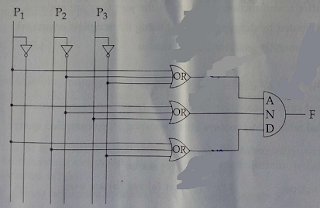151. In 8085 microprocessor, what is the output of following program?LDA 8000HMVI B, 30HADD BSTA 8001H a. Read a number from input port and store it in memory b. Read a number from input device with address 8000H and store it in memory at location 8001H c. Read a number from memory at location 8000H and store it in memory location 8001H d. Load A with data from input device with address 8000H and display it on the output device with address 8001H
 Answer: (d).Load A with data from input device with address 8000H and display it on the output device with address 8001H

 152. The Boolean function with the following Karnaugh map is : a. (A + C).D + B b. (A + B).C + D c. (A + D).C + B d. (A + C).B + D
 Answer: (a).(A + C).D + B

 153. The Octal equivalent of the binary number 1011101011 is: a. 7353 b. 1353 c. 5651 d. 5657

 154. The output of the following combinational circuit is F. The value of F is :a. P1+P2’P3 b. P1+P2’P3’ c. P1+P2P3’ d. P1’+P2P3

 155. In 8085 microprocessor which of the following flag(s) is (are) affected by an arithmetic operation? a. AC flag Only b. CY flag Only c. Z flag Only d. AC, CY, Z flags
 Answer: (d).AC, CY, Z flags

 156. In 8085 microprocessor the address bus is of .................... bits. a. 4 b. 8 c. 16 d. 32

 157. In the architecture of 8085 microprocessor match the following:List - I(a) Processing unit(b) Instruction unit(c) Storage and Interface unitList - II(i) Interrupt(ii) General purpose Register(iii) ALU(iv) Timing and ControlCode : (a) (b) (c) a. (iv) (i) (ii) b. (iii) (iv) (ii) c. (ii) (iii) (i) d. (i) (ii) (iv)
 Answer: (b).(iii) (iv) (ii)

 158. Which of the following addressing mode is best suited to access elements of an array of contiguous memory locations? a. Indexed addressing mode b. Base Register addressing mode c. Relative address mode d. Displacement mode